### Home > CALC > Chapter 1 > Lesson 1.2.5 > Problem1-89

1-89.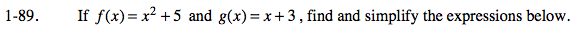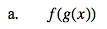f(g(x)) = f(x + 3) = ______________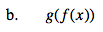Refer to hint (a).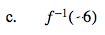Even though f −1(x) is the inverse of f(x), you do not need to find the equation of the inverse. Simply evaluate 6 as the y-value and solve for x.

6 = f(x)
6 = x2 + 5
1 = x2
x = ± 1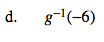Refer to hint (c).